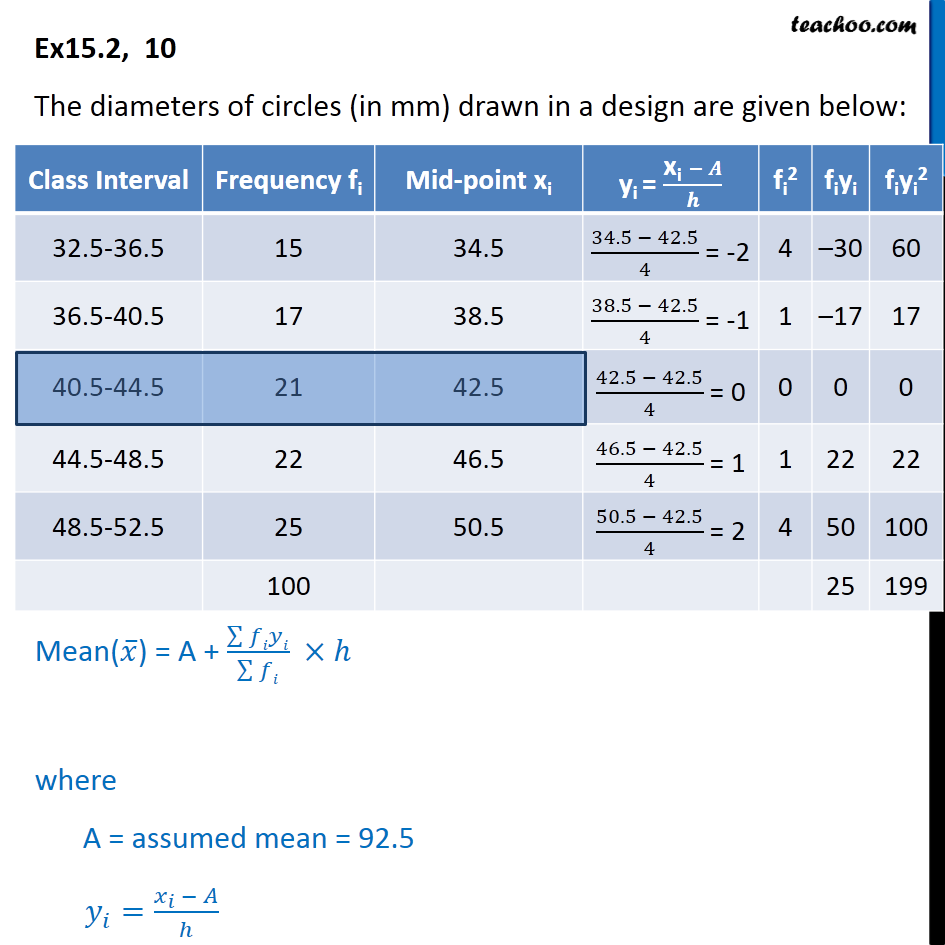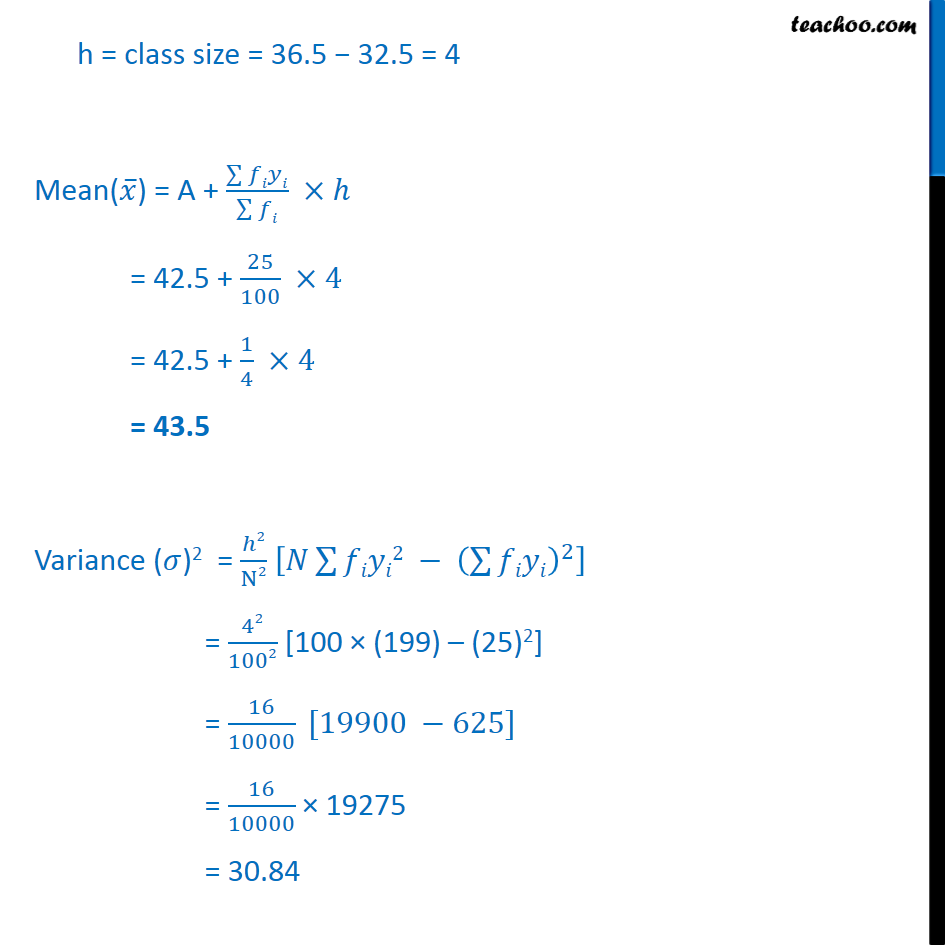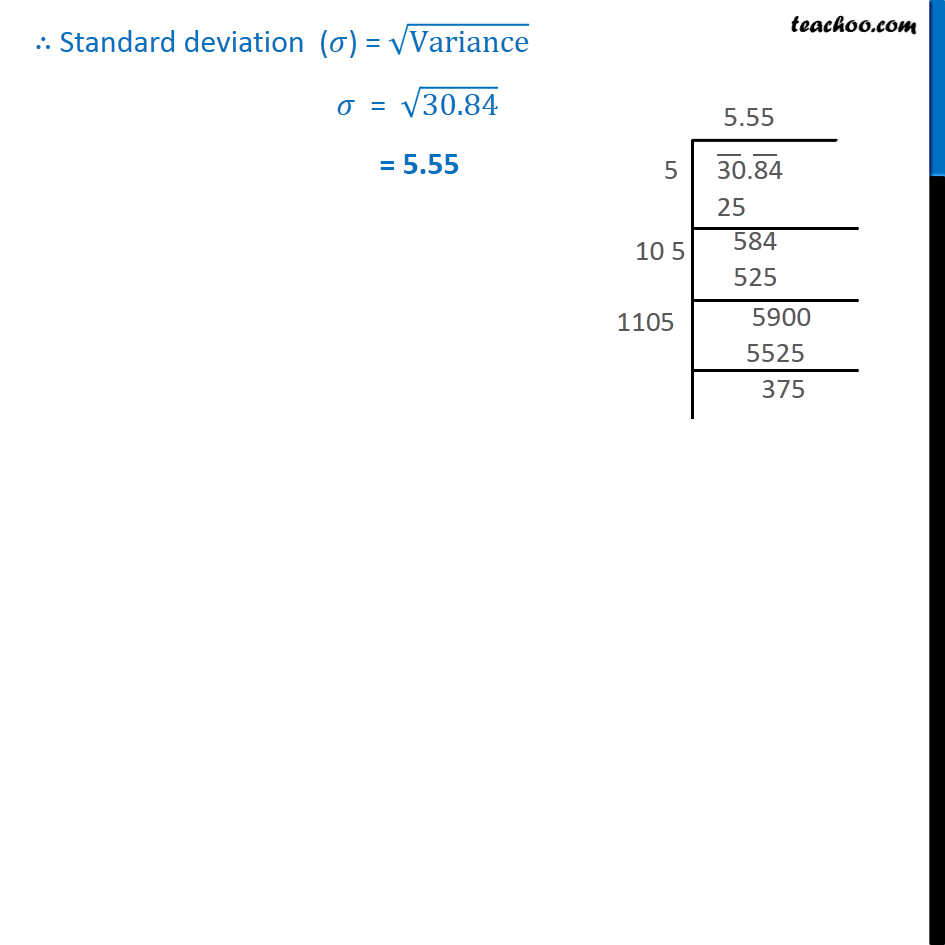Ex 13.2

Chapter 13 Class 11 Statistics
Serial order wiseLearn in your speed, with individual attention - Teachoo Maths 1-on-1 Class

### Transcript

Ex 13.2, 10 The diameters of circles (in mm) drawn in a design are given below: h = class size = 36.5 − 32.5 = 4 Mean(𝑥 ̅) = A + (∑▒𝑓𝑖𝑦𝑖)/(∑▒𝑓𝑖) ×ℎ = 42.5 + 25/100 ×4 = 42.5 + 1/4 ×4 = 43.5 Variance (𝜎)2 = ℎ2/N2 [𝑁∑▒𝑓𝑖𝑦𝑖2 − (∑▒𝑓𝑖𝑦𝑖)^2 ] = 42/1002 [100 × (199) – (25)2] = 16/10000 [19900 −625] = 16/10000 × 19275 = 30.84 ∴ Standard deviation (𝜎) = √Variance 𝜎 = √30.84 = 5.55